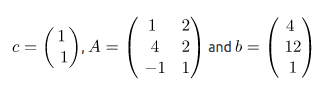{{announcement.body}}
{{announcement.title}}

# Linear Programming With Python

DZone 's Guide to

# Linear Programming With Python

### In this post, we use the example of linear programming to show the scientific and mathematical applications of the Python language.

· Big Data Zone ·
Free Resource

Comment (0)

Save
{{ articles.views | formatCount}} Views

A linear programming problem may be defined as the problem of maximizing or minimizing a linear function subject to linear constraints. The constraints may be equalities or inequalities.

Here is a simple example: find numbers x1 and x2 that maximize the sum x1 + x2 subject to the constraints x1 ≥ 0, x2 ≥ 0, and

``````x1 + 2x2 ≤ 4
4x1 + 2x2 ≤ 12
−x1 + x2 ≤ 1``````

The first two constraints, x1 ≥ 0 and x2 ≥ 0 are called nonnegativity constraints. The other constraints are then called the main constraints. The function to be maximized (or minimized) is called the objective function. Here, the objective function is x1 + x2.

Two classes of problems, called here the standard maximum problem and the standard minimum problem, play a special role. In these problems, all variables are constrained to be nonnegative, and all main constraints are inequalities.

## Standard Maximum Problem

We are given an m-vector,an n-vector,and an m x n matrix of real numbersFind an n-vector,to maximizesubject to the constraintsand### Standard Minimum Problem

Find an n-vector,to minimizesubject to the constraintsand#### Example

The example at the beginning of this post corresponds to the standard maximum problem, where:The solutions are:

``````from pulp import *
from fractions import Fraction

prob = LpProblem("Example of standard maximum problem",LpMaximize)

# nonnegativity constraints
x1=LpVariable("x1",0)
x2=LpVariable("x2",0)

# objective function
prob += x1 + x2, "Maximum value of x1 + x2"

# main constraints
prob += x1 + 2*x2 <= 4, "constraint 1"
prob += 4*x1 + 2*x2 <= 12, "constraint 2"
prob += -x1 + x2 <= 1, "constraint 3"

# The problem is solved using PuLP's choice of Solver
prob.solve()

# status of the solution
print(f"Status: {LpStatus[prob.status]}")

for v in prob.variables():
print(f"{v.name} = {str(Fraction(v.varValue).limit_denominator())}")

# maximum value of the objective function
print(f"max (x1 + x2) = {str(Fraction(value(prob.objective)).limit_denominator())}")``````
``````Status: Optimal
x1 = 8/3
x2 = 2/3
max (x1 + x2) = 10/3``````

The importance of the standard problem derives from the fact that all linear programming problems can be converted to standard form.

What is more, a minimum problem can be changed to a maximum problem by:

• multiplying the objective function by -1
• multiplying the constraint inequalities by -1 and reversing the inequalities

Let's prove it by changing the previous example to a minimum problem:

``````from pulp import *
from fractions import Fraction

prob = LpProblem("Example of standard minimum problem",LpMinimize)

# nonnegativity constraints
x1=LpVariable("x1",0)
x2=LpVariable("x2",0)

# objective function
prob += -x1 - x2, "Minimum value of -x1 - x2"

# main constraints
prob += -x1 - 2*x2 >= -4, "constraint 1"
prob += -4*x1 - 2*x2 >= -12, "constraint 2"
prob += x1 - x2 >= -1, "constraint 3"

# The problem is solved using PuLP's choice of Solver
prob.solve()

# status of the solution
print(f"Status: {LpStatus[prob.status]}")

for v in prob.variables():
print(f"{v.name} = {str(Fraction(v.varValue).limit_denominator())}")

# maximum value of the objective function
print(f"min (-x1 - x2) = {str(Fraction(value(prob.objective)).limit_denominator())}")``````
``````Status: Optimal
x1 = 8/3
x2 = 2/3
min (-x1 - x2) = -10/3``````

### Duality

For every linear program there is a dual linear program. The dual of the standard maximum problem (as defined above) is defined to be the standard minimum problem:

Find an m-vector,to minimizesubject to the constraintsandTherefore, the dual problem of the initial example is:

``````from pulp import *
from fractions import Fraction

prob = LpProblem("Dual problem",LpMinimize)

# nonnegativity constraints
y1=LpVariable("y1",0)
y2=LpVariable("y2",0)
y3=LpVariable("y3",0)

# objective function
prob += 4*y1 + 12*y2 + y3, "Minimum value of 4*y1 + 12*y2 + y3"

# main constraints
prob += y1 + 4*y2 - y3 >= 1, "constraint 1"
prob += 2*y1 + 2*y2 + y3 >= 1, "constraint 2"

# The problem is solved using PuLP's choice of Solver
prob.solve()

# status of the solution
print(f"Status: {LpStatus[prob.status]}")

for v in prob.variables():
print(f"{v.name} = {str(Fraction(v.varValue).limit_denominator())}")

# maximum value of the objective function
print(f"min (4*y1 + 12*y2 + y3) = {str(Fraction(value(prob.objective)).limit_denominator())}")``````
``````Status: Optimal
y1 = 1/3
y2 = 1/6
y3 = 0
min (4*y1 + 12*y2 + y3) = 10/3``````

As the reader must have noticed, the number of main constraints of the standard problem equals the number of nonnegative constraints of its dual. According to the Equilibrium Theorem, strict inequality in a constraint in a standard problem implies that the complementary constraint in the dual is satisfied with equality, and viceversa.

Let's verify that assertion:

``````print([x1 + 2*x2 == 4, 4*x1 + 2*x2 == 12, -x1 + x2 == 1] == [not x for x in [y1.varValue == 0, y2.varValue == 0, y3.varValue == 0]])
print([y1 + 4*y2 - y3 == 1, 2*y1 + 2*y2 + y3 == 1] == [not x for x in [x1.varValue == 0, x2.varValue == 0]])``````
``````True
True``````

Although, in this case, the value of the objective function corresponding to the vectors x and is the same, this is not always true. It can be proved that, when this is true, the solution is optimal for both problems.

More generally, the values of the standard objective function (compatible with its constraints) are always ≤ than the values of the dual objective function (compatible with its constraints). In other words, the dual problem provides an upper bound to the optimal value of the original problem.

Topics:
big data, linear programming, python tutorial, scientific python

Comment (0)

Save
{{ articles.views | formatCount}} Views

Published at DZone with permission of Francisco Alvarez , DZone MVB. See the original article here.

Opinions expressed by DZone contributors are their own.